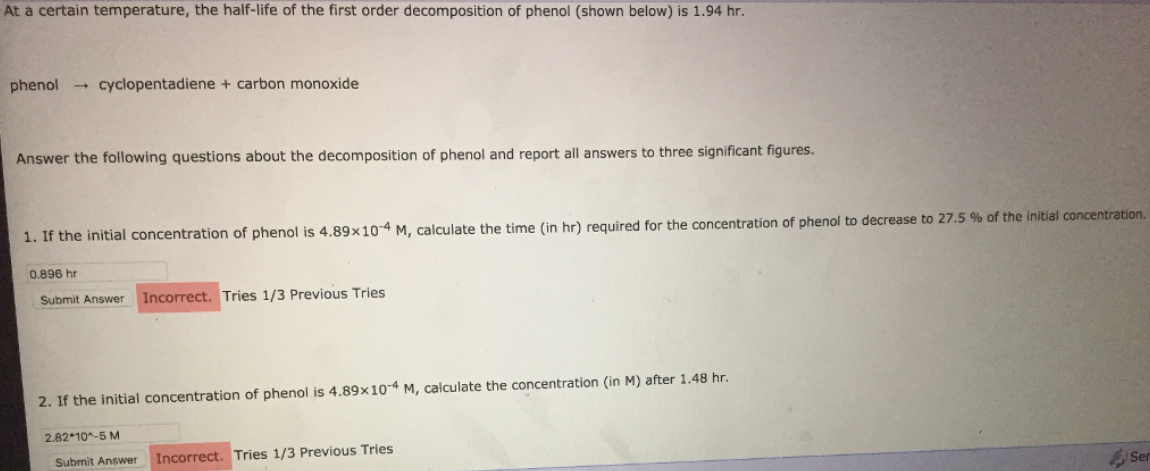# Problem: At a certain temperature, the half-life of the first order decomposition of phenol (shown below) is 1.94 hr.phenol → cyclopentadiene + carbon monoxideAnswer the following questions about the decomposition of phenol and report all answers to three significant figures.1. If the initial concentration of phenol is 4.89 times 10-4 M, calculate the time (in hr) required for the concentration of phenol to decrease to 27.5% of the initial concentration. 0.896 hr2. If the initial concentration of phenol is 4.89 times 10-4 M, calculate the concentration (in M) after 1.48 hr. 2.82*10-5 M

###### FREE Expert Solution
89% (442 ratings)###### Problem Details

At a certain temperature, the half-life of the first order decomposition of phenol (shown below) is 1.94 hr.

phenol → cyclopentadiene + carbon monoxide

Answer the following questions about the decomposition of phenol and report all answers to three significant figures.

1. If the initial concentration of phenol is 4.89 times 10-4 M, calculate the time (in hr) required for the concentration of phenol to decrease to 27.5% of the initial concentration. 0.896 hr

2. If the initial concentration of phenol is 4.89 times 10-4 M, calculate the concentration (in M) after 1.48 hr. 2.82*10-5 M